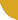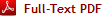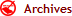|Twitter |Facebook |Google+ |VKontakte | LinkedIn|International Journal of Innovation and Scientific Research
ISSN: 2351-8014
 Monday 22 July 2019

# Connect with IJISR

•••••••# Analysis of the determinants of the mode of disposal of municipal solid waste in the main urban centers of the North Benin: case of the cities of Parakou, Djougou, Kandi and Malanville

[ Analyse des déterminants du mode d'évacuation des déchets solides ménagers dans les principaux centres urbains du nord-Bénin : cas des villes de Parakou, Djougou, Kandi et Malanville ]

Volume 20, Issue 2, February 2016, Pages 428–444Sanni BABIO and Christophe S. HOUSSOU

Original language: French

Copyright © 2016 ISSR Journals. This is an open access article distributed under the Creative Commons Attribution License, which permits unrestricted use, distribution, and reproduction in any medium, provided the original work is properly cited.

# Abstract

The determinants that influence the mode of disposal of household solid waste were studied in the cities of Parakou, Djougou, Kandi and Malanville in northern Benin. Sex, age, educational level, marital status, income, household size, socio-professional category, type of neighborhood, type of habitat, cities are the variables taken into account and analyzed by the Chi2 test and logistic regression. The results showed little overall trend varies from one city to another household to evacuate their garbage directly in nature (χ2 = 1.188; DF = 3; P-Value = 0.756> 0.05). Moreover, no statistically significant association was detected between the mode of waste disposal and sex (χ2 = 1.267; DF = 3; P-Value = 0.737> 0.05), age of household heads (χ2 = 2.868; DF = 4; P-Value = 0.580> 0.05), marital status (χ2 = 7.710, DF = 9; P-Value = 0.564> 0.05); household size (χ2 = 4.688; DF = 3; P-Value = 0.196> 0.05). In the cities studied, only in Parakou, χ2 = 18.275; DF = 7; P-Value = 0.011 <0.05 and Djougou, χ2 = 17.108; DF = 9; P-Value = 0.047 <0.05, very significant links were found between the mode of waste disposal and the occupational category of household heads. Note also that no relationship was statistically observed between the mode of waste disposal and the level of heads of household income in Djougou, χ2 = 3.909; DF = 4; P-Value = 0.418> 0.05); Kandi, χ2 = 2.826; DF = 3; P-Value = 0.419> 0.05) and Malanville, χ2 = 2.482; DF = 3; P-Value = 0.479> 0.05). But in the four cities, 100% very highly significant association was observed between link waste disposal and education level of household heads at the global level (χ2 = 28.328; DF = 3; P-Value = 0.000 <0.05). Logistic regression analysis also revealed a strong correlation between educational level (χ2 = 9.439; DF = 3; P-Value = 0.024 <0.05 and mode of waste disposal in North Benin.

Author Keywords: Determinants, mode, disposal, waste, North-Benin.

# Abstract: (french)

Les déterminants qui influencent le mode d'évacuation des déchets solides ménagers ont été étudiés dans les villes de Parakou, Djougou, Kandi et Malanville au nord du Bénin. Le sexe, l'âge, le niveau d'instruction, le statut matrimonial, le revenu, la taille du ménage, la catégorie socio-professionnelle, le type de quartier, le type d'habitat, les villes sont les variables prises en compte et analysées par le test de Khi2 et la régression logistique. Les résultats ont révélé une tendance globale peu variable d'une ville à une autre des ménages à faire évacuer leurs ordures directement dans la nature (χ2= 1,188; DF = 3; P-Value = 0,756 >0,05). Par ailleurs, aucun lien statistiquement significatif n'a été décelé entre le mode d'évacuation des déchets et le sexe (χ2 = 1,267; DF = 3; P-Value = 0,737>0,05), l'âge des chefs de ménages (χ2 = 2,868; DF = 4; P-Value = 0,580>0,05), le statut matrimonial (χ2= 7,710; DF = 9; P-Value = 0,564>0,05) ; la taille des ménages (χ2 = 4,688; DF = 3; P-Value = 0,196>0,05). C'est à Parakou, χ2= 18,275; DF = 7; P-Value = 0,011<0,05 et à Djougou, χ2 = 17,108; DF = 9; P-Value = 0,047<0,05, que des liens très significatifs ont été décelés entre le mode d'évacuation des déchets et la catégorie socio-professionnelle des chefs de ménages. Notons aussi qu'aucune relation n'a été statistiquement observée entre le mode d'évacuation des déchets et le niveau de revenu des chefs de ménages à Djougou, χ2= 3,909; DF = 4; P-Value = 0,418>0,05) ; à Kandi, χ2= 2,826; DF = 3; P-Value = 0,419>0,05) et à Malanville, χ2 = 2,482; DF = 3; P-Value = 0,479>0,05). Mais dans les quatre villes, à 100 % un lien associatif très hautement significatif a été observé entre l'évacuation des déchets et le niveau d'instruction des chefs de ménages au niveau global (χ2 = 28,328; DF = 3; P-Value = 0,000 <0,05). L'analyse de régression logistique a par ailleurs révélé une très forte corrélation entre le niveau d'instruction (χ2= 9,439; DF = 3; P-Value = 0,024<0,05 et le mode d'évacuation des déchets au nord-Bénin.

Author Keywords: Déterminants, mode, évacuation, déchets, nord-Bénin.